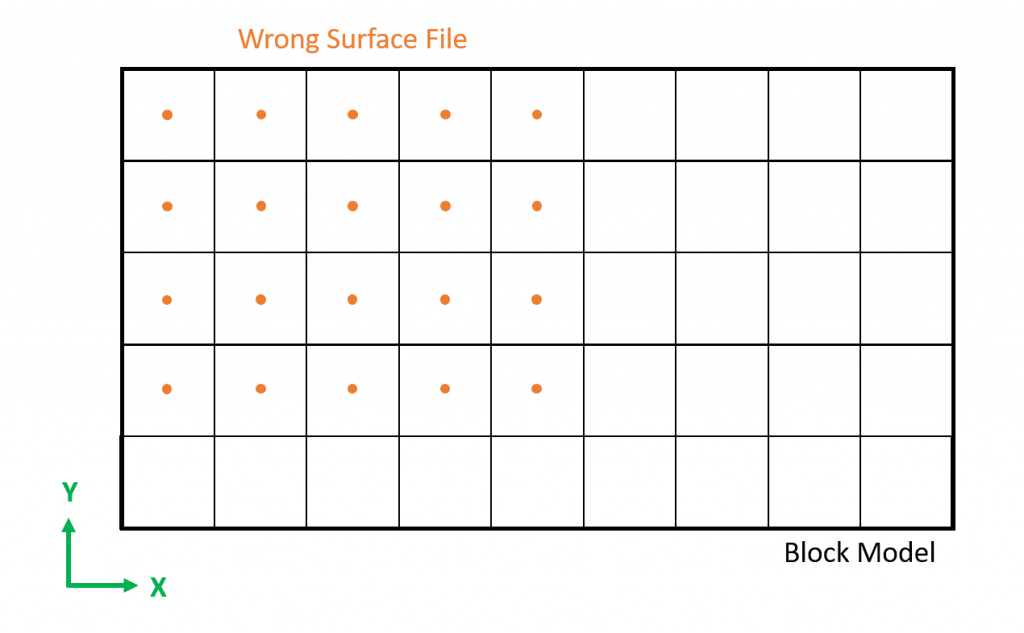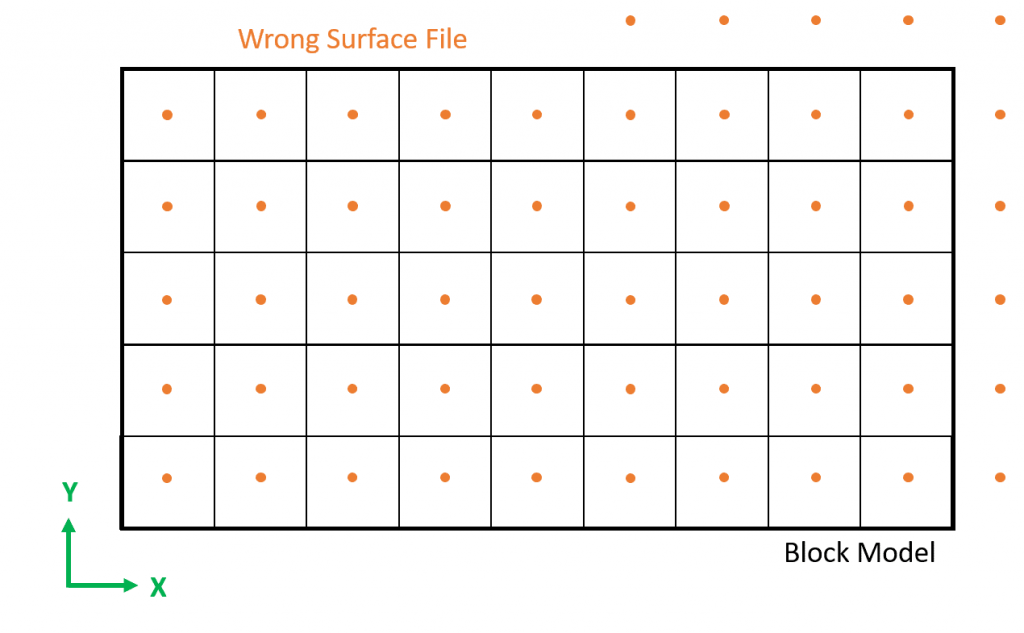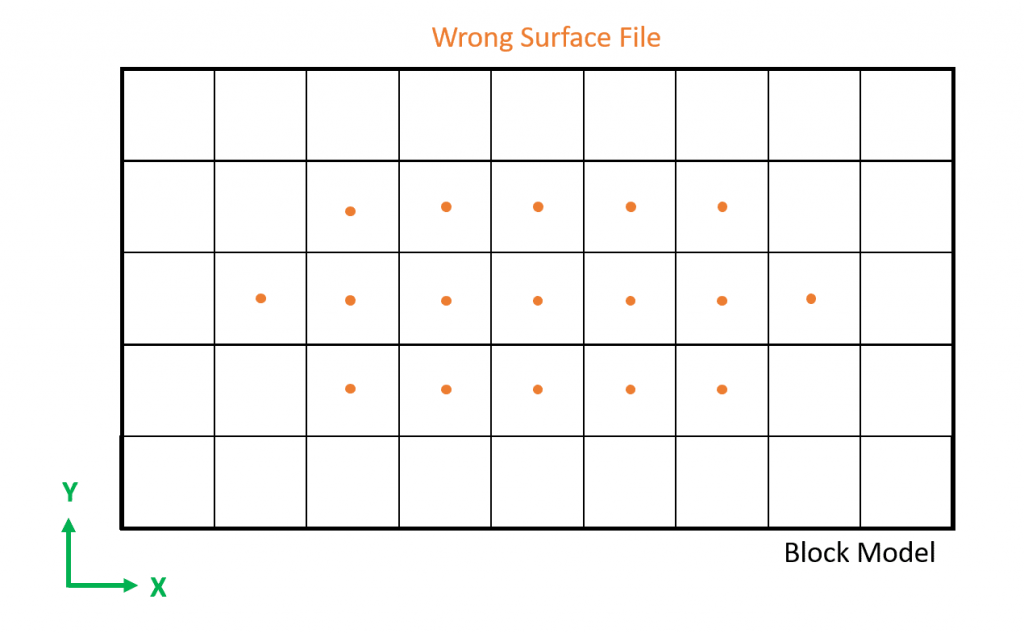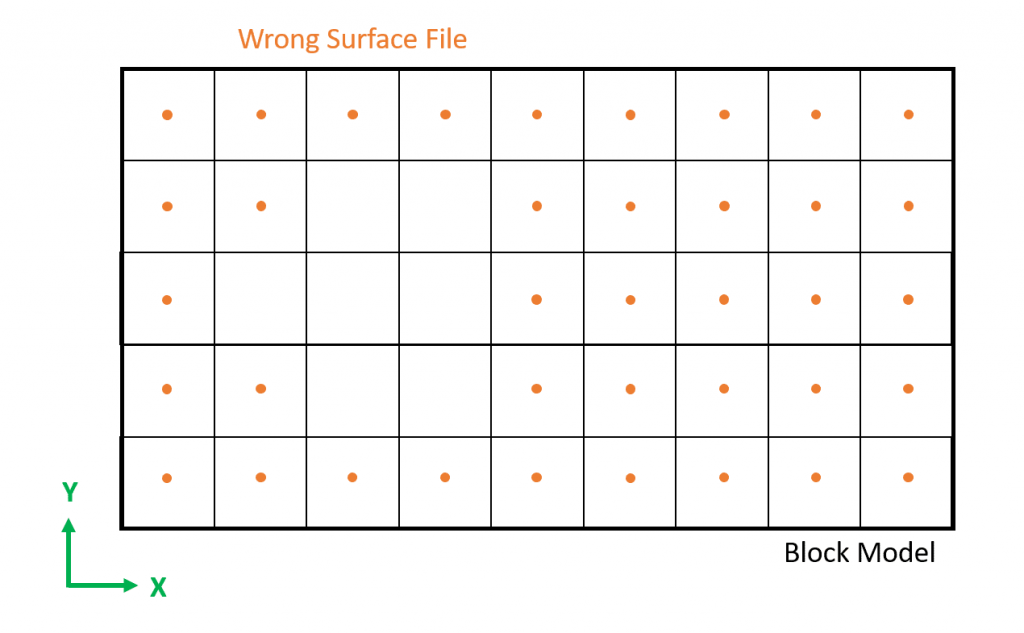#### MiningMath

Easily integratable to your preferred package through CSV files

# Validating Surfaces

Estimated reading: 4 minutes 496 views

## Introduction

The best way to generate surfaces is by using a topography surface created by MiningMath, which is created after the Data Validation, then manipulating only the Z coordinates. Make sure to meet all the surface requirements disclosed here.

Surface Requirements
• Headers must be named as X, Y, and Z. These files have to obey an ascending value order in each one of the axes.

• Same size of the block model, item 1.1 of this page explains it.

• Its points must be aligned with blocks' centroidsitems 1.1 and 1.2 help you to understand it.

• Defined as a grid of points, the visual validation, item 1.2 shows it.

• To be in the CSV format.

## Surface and Block Model boundaries

By using the values of a Marvin Deposit file in Figure 2, we find the Block model centroids boundaries which begin at XMin=15; YMin=15 and has the maximum centroid value of XMax=5,295YMax=8,265, which could be confirmed by checking the topography generated by MiningMath.

Then, its time to search for your surface limits, at this example the file chosen was “Surface-RM-offset-300m”. The easy way to find it, is by filtering the axis values, as shown in Figure 3, which disclosed XMin= 15; YMin=15 and XMax= 1,815 and YMax= 1,785. Therefore, even though this file was also based on Marvin Deposit, it is not possible to use it since the surface smaller than the block model in place, which means that it does not have the same size as the block model file.

It is always worthwhile to check the limits of the designed surface (Figures 4 to 8) if we face an error. Remember that everything, even elevations, must be in the boundaries of the block model and check the recommendations for your surface.Figure 4: Surface (grid of points) smaller than the block model.Figure 6: Surface (grid of points) bigger than the block model.Figure 5: Surface (grid of points) smaller than the block model.Figure 7: Surface (grid of points) unaligned with the centroids.Figure 8: Correct Surface (grid of points) aligned with the centroids and same block model size.

## Maximum boundaries formula

The centroids maximum limit by using the equation for each axis:

Maximum centroid value = OX + (NX*DX)-(DX/2)

Where:

• OX is the origin of the X-axis;

• DX is the block dimension of the X-axis;

• NX is the number of blocks in the X-axis.

Note: (DX/2) is related to the distance which should be summed on the origin to find the centroid of the first block, or reduced at the block model limit to find the last centroid, since origins are based on the corner of the block model.

## Numeric and visual validation

The following video presents how to validate surfaces numerically and visually. This initial verification is what enables you to understand what might be happening and where the error is. The example used regards to the message: “Error parsing surface: Coordinates aren’t properly spaced” but it fits any case where the surfaces used are causing a problem, especially when a red box error shows up.

In this case, as there are a lot of values that do not match the correct ones for X and Y, the quicker and easier way to fix it is restarting from the beginning.

Video 1: Validating surfaces.

## Protected: Imperial system

There is no excerpt because this is a protected post.

## In-depth MiningMath

This tutorial provides a detailed guidance to the pages in the knowledge base fo...

## Theory Validation

MiningMath’s results are only possible due to its proprietary Math Program...

## Guaranteed Solutions

Multiple, complex constraints increase the likelihood of not finding or not exis...

## MiningMath Uniqueness

MiningMath allows mining managers to improve their strategic analysis through ri...

## Time Limit

It is possible to indicate a time limit in hours before running a scenario in th...

## Must read articles

In order to take the maximum of MiningMath’s Optimization we recommend this fl...

## Formatting the Block Model

The main focus here is on the requirements. Try to pay attention to the header...